# PSAT Math : Statistics

## Example Questions

### Example Question #21 : Statistics

Daria plays darts often. She scores 25 points 1/5 of the time she plays. She also scores 50 points with probability 3/5, and 0 points with probability 1/5. What is the average number of points Daria scores when she plays darts?

25

20

35

40

30

35

Explanation:

To find the average number of points, we need to multiply the points by their corresponding weights and sum them up.

Average = 1/5 * 25 + 3/5 * 50 + 1/5 * 0 = 35.

### Example Question #23 : Statistics

Mrs. Hovsepian teaches 5 different math classes.

The first class contains 42 student. The second class has 38 students. The third class has 67 students. The fourth class has 25 students. The fifth class has 28 students.

What is the mean number of students per class?

42

40

47

38

43

40

Explanation:

To get the mean we must add up all of the students and divide by the number of classes. When we add them all up there are 200 total students.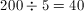### Example Question #33 : Statistics

Find the arithmetic mean of the following set of numbers: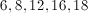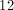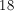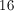Explanation:

The mean is adding all the numbers then dividing by the number of items.

In this case we have,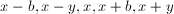where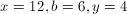When this happens, the average is### Example Question #34 : Statistics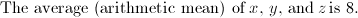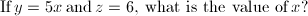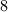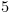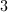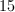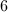Explanation:

Arithmetic mean: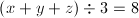given: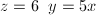Solve: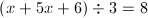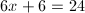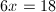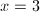### Example Question #31 : Statistics

A class consists of 10 students. The teacher wrote the test and expects the class to have an average score of at least 80. The average score of 9 people was calculated and it was 78. What score does the last person have to get in order to reach the teacher's standard?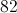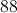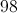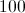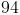Explanation:

The total score of those 9 students is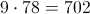.

The standard score for the entire class was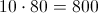.

Therefore, the last person needs a score of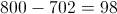.

### Example Question #31 : Statistics

What is the average (mean) of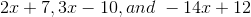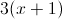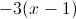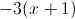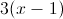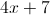Explanation:

By adding all of the expressions we get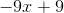which averages to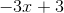,

which will simplify to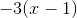.

### Example Question #31 : How To Find Arithmetic Mean

The mean of a series of 6 numbers (11, 12, 14, 17, 18, x) is 15. Solve for x.

19

18

17

16

18

Explanation:

If the mean of 6 numbers is 15, that implies that the sum of the numbers is equal to 90 (6 x 15).

Therefore,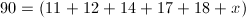or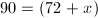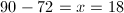### Example Question #36 : Statistics

A police officer walked into a room full of suspects and turn out their pockets.  The chart below shows the number of coins each man had.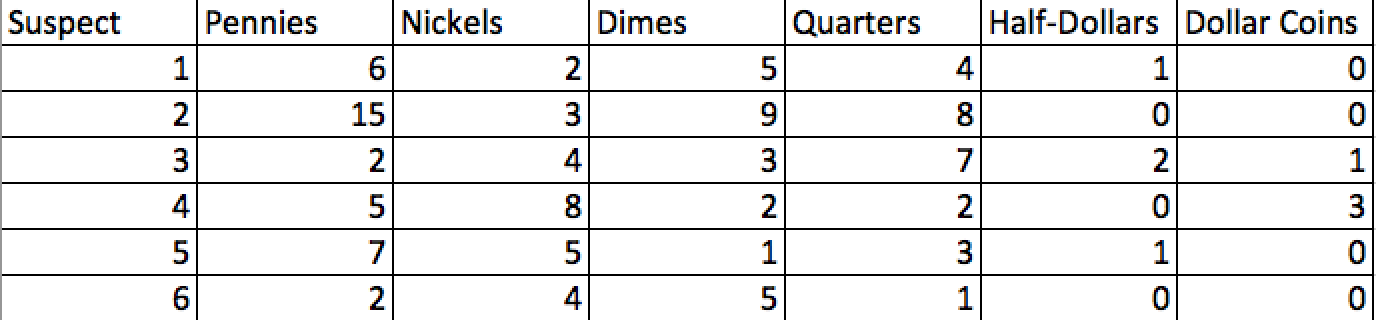The police claim the man with the amount of money closest to the arithmetic mean of the group is guilty.  Who is it?

Suspect 4

Suspect 3

Suspect 2

Suspect 1

Suspect 6

Suspect 2

Explanation: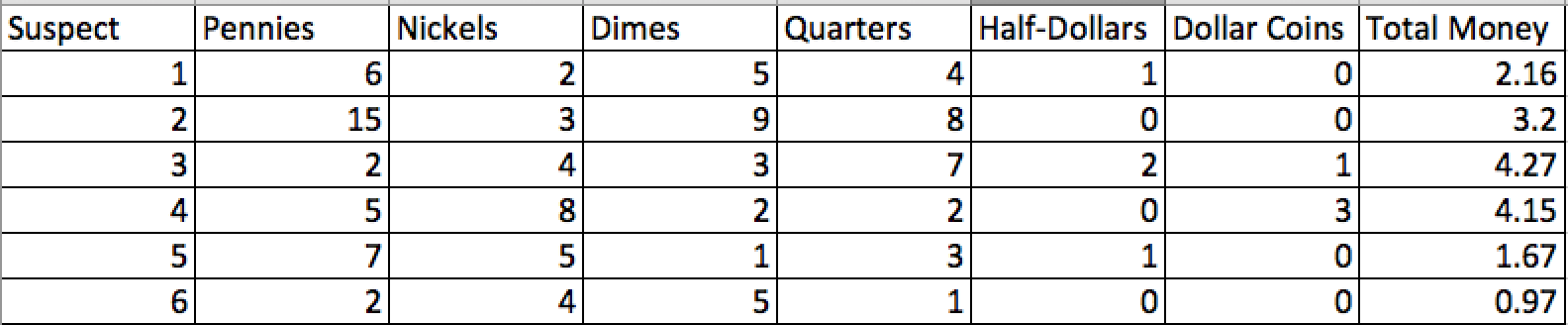Summing total money and dividing by the number of suspects gives us an average of approximately \$2.73.  By comparing it to the amounts held by the suspects, we can see that Suspect #2 is guilty.

### Example Question #32 : Statistics

The average temperature for 6 days in July is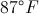. If on the seventh day, the temperature is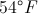, what is the average temperature over those seven days?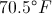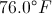There is not enough information to answer this question.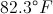Explanation:

In order to calculate mean, we can act as though the temperature was exactlyfor the first six days andon the seventh day. The mean can be expressed: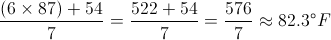### Example Question #37 : Statistics

If the average  of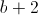is equal to the average of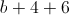. What is the value of?

10

16

8

20

14

14

Explanation: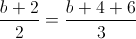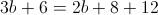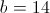### All PSAT Math Resources